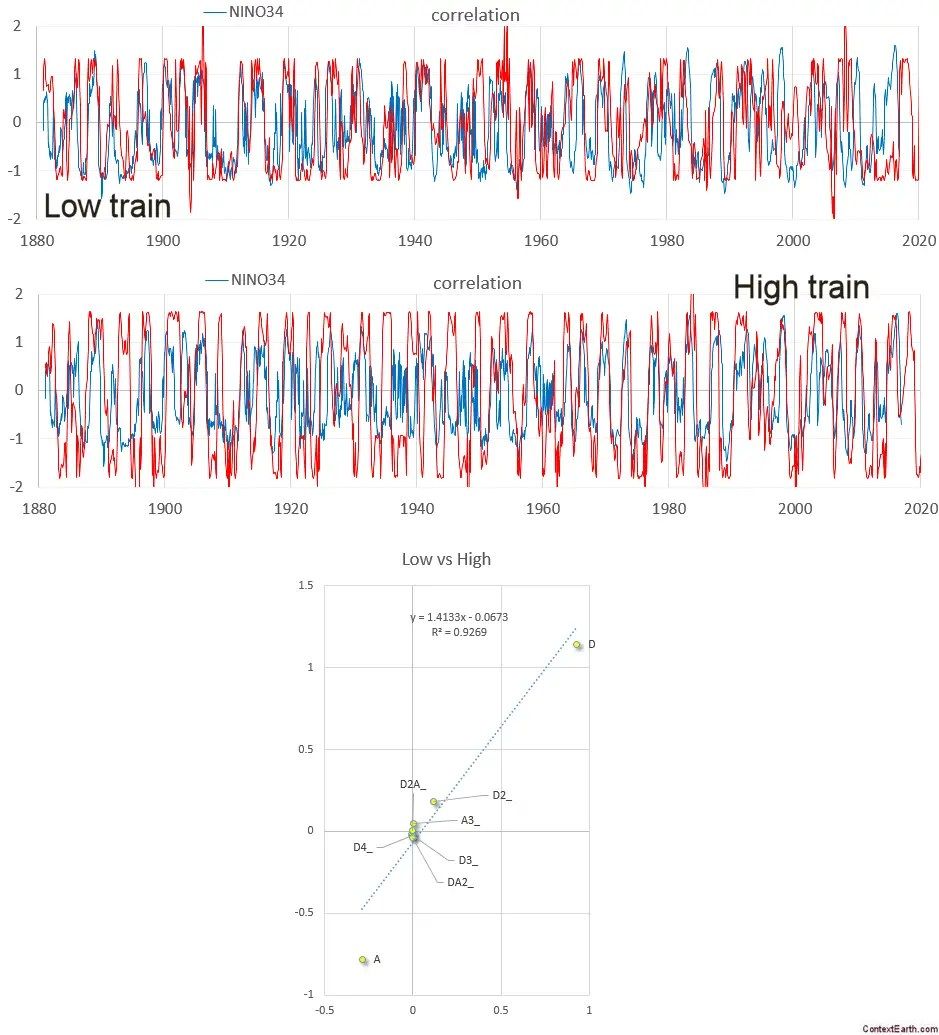# Improved Solver Target Error Metric

In addition to the algorithm used for solving optimization problems, an important criteria is the form of the metric used to minimize the error or maximize the similarity between data and model.

The commonly used forms such as error variance (i.e. mean squared error) have issues related to how well they can navigate the search space. Other forms such as correlation coefficient (CC) often work better, but at the expense of losing track of scale.  This indicates that CC is better at matching the general characteristics of a specific shape than a pure error criteria. And if weighted, it can deal with noisy intervals.

In fact, the symbolic reasoner Eureqa features a proprietary metric referred to as a hybrid correlation coefficient.  From my experiences with the tool, hybrid version does qualitatively work better.

So in my quest to find an alternative metric, I came up with something related to the Cosine Similarity (CS) measure. As defined, CS is not that different from Pearson’s correlation coefficient as it does not subtract the mean. But with a slight modification it’s an excellent “starter” metric for initial exploration.

The new metric is essentially a +/- excursion matching criteria (EMC), which is important for a behavior as cyclically erratic about the origin as ENSO.

The algorithm for the EMC can be described as a ratio of two factors. The numerator is the sum of the multiplications of the model and data values. The denominator is the normalizing factor, which is the sum of the multiplication of the absolute values of each value.$EMC = frac{sum x_i cdot y_i}{sum |x_i| cdot |y_i|}$

The resulting metric ranges from -1 to 1, with 1 being a perfect sign excursion matching, and -1 if all excursions had the sane magnitude but were reversed in sign.

This of course is not a perfect criteria as it will tend to force the minimal excursions to zero while maximizing the maximum excursions, instead of first normalizing them as the true CS does.

The evidence to how well it works is mainly based on observations in massive reductions in search time. For ENSO model optimization search, the EMC reduces the time it takes to get in the ballpark by 100×, so what could take an hour reduces to about a minute of computational time. It is important not to let it overfit, so wait until the metric starts to slow in its improvement before stopping the search and switching to the CC metric for the final stages optimization.

As it is so fast I have been using it for minimally filtered ENSO time series, where I can start from minimally seed sets of parameters. This gives more confidence that results are not correlated from one search optimization run to the next.The EMC is therefore a great metric for randomizing searches. I can imagine using it in a scenario with different initialized seed values and then waiting a fixed time to return an interim solution, and then using the best of these in a more refined CC search.

Why it works so well is something I am still trying to explain. It is a more efficient computation than CC, but that is not enough to explain 100x.